• 针对目前反向计算模型还无法实现对建筑室内边界对流换热量进行反向计算这一制约性差距，采用温度贡献率方法，将边界对流换热量与室内测点温度之间表示成因果关系的温度贡献因子矩阵，基于计算流体力学，将最小二乘与...
• 反向计算过程中，对流扩散方程的对流项一方面具有强烈的方向性，意味着上游信息通过对流项向下游传递；另一方面由于是逆向计算，因此计算时下游信息也需要通过对流项传递到上游。此外与顺时计算不同，扩散项在反向...
• 个税计算工具网上有的是 这个工具可以通过所交的个人所得税反向计算 可以得到所交个税的工资 这个功能比较有意思！
正向传播、反向传播和计算图
前面几节里我们使用了小批量随机梯度下降的优化算法来训练模型。在实现中，我们只提供了模型的正向传播（forward propagation）的计算，即对输入计算模型输出，然后通过autograd模块来调用系统自动生成的backward函数计算梯度。基于反向传播（back-propagation）算法的自动求梯度极大简化了深度学习模型训练算法的实现。本节我们将使用数学和计算图（computational graph）两个方式来描述正向传播和反向传播。具体来说，我们将以带

L

2

L_2

范数正则化的含单隐藏层的多层感知机为样例模型解释正向传播和反向传播。
正向传播
正向传播是指对神经网络沿着从输入层到输出层的顺序，依次计算并存储模型的中间变量（包括输出）。为简单起见，假设输入是一个特征为

x

∈

R

d

\boldsymbol{x} \in \mathbb{R}^d

的样本，且不考虑偏差项，那么中间变量

z

=

W

(

1

)

x

,

\boldsymbol{z} = \boldsymbol{W}^{(1)} \boldsymbol{x},

其中

W

(

1

)

∈

R

h

×

d

\boldsymbol{W}^{(1)} \in \mathbb{R}^{h \times d}

是隐藏层的权重参数。把中间变量

z

∈

R

h

\boldsymbol{z} \in \mathbb{R}^h

输入按元素运算的激活函数

ϕ

\phi

后，将得到向量长度为

h

h

的隐藏层变量

h

=

ϕ

(

z

)

.

\boldsymbol{h} = \phi (\boldsymbol{z}).

隐藏层变量

h

\boldsymbol{h}

也是一个中间变量。假设输出层参数只有权重

W

(

2

)

∈

R

q

×

h

\boldsymbol{W}^{(2)} \in \mathbb{R}^{q \times h}

，可以得到向量长度为

q

q

的输出层变量

o

=

W

(

2

)

h

.

\boldsymbol{o} = \boldsymbol{W}^{(2)} \boldsymbol{h}.

假设损失函数为

ℓ

\ell

，且样本标签为

y

y

，可以计算出单个数据样本的损失项

L

=

ℓ

(

o

,

y

)

.

L = \ell(\boldsymbol{o}, y).

根据

L

2

L_2

范数正则化的定义，给定超参数

λ

\lambda

，正则化项即

s

=

λ

2

(

∥

W

(

1

)

∥

F

2

+

∥

W

(

2

)

∥

F

2

)

,

s = \frac{\lambda}{2} \left(\|\boldsymbol{W}^{(1)}\|_F^2 + \|\boldsymbol{W}^{(2)}\|_F^2\right),

其中矩阵的Frobenius范数等价于将矩阵变平为向量后计算

L

2

L_2

范数。最终，模型在给定的数据样本上带正则化的损失为

J

=

L

+

s

.

J = L + s.

我们将

J

J

称为有关给定数据样本的目标函数，并在以下的讨论中简称目标函数。
正向传播的计算图
我们通常绘制计算图来可视化运算符和变量在计算中的依赖关系。图3.6绘制了本节中样例模型正向传播的计算图，其中左下角是输入，右上角是输出。可以看到，图中箭头方向大多是向右和向上，其中方框代表变量，圆圈代表运算符，箭头表示从输入到输出之间的依赖关系。反向传播
反向传播指的是计算神经网络参数梯度的方法。总的来说，反向传播依据微积分中的链式法则，沿着从输出层到输入层的顺序，依次计算并存储目标函数有关神经网络各层的中间变量以及参数的梯度。对输入或输出

X

,

Y

,

Z

\mathsf{X}, \mathsf{Y}, \mathsf{Z}

为任意形状张量的函数

Y

=

f

(

X

)

\mathsf{Y}=f(\mathsf{X})

和

Z

=

g

(

Y

)

\mathsf{Z}=g(\mathsf{Y})

，通过链式法则，我们有

∂

Z

∂

X

=

prod

(

∂

Z

∂

Y

,

∂

Y

∂

X

)

,

\frac{\partial \mathsf{Z}}{\partial \mathsf{X}} = \text{prod}\left(\frac{\partial \mathsf{Z}}{\partial \mathsf{Y}}, \frac{\partial \mathsf{Y}}{\partial \mathsf{X}}\right),

其中

prod

\text{prod}

运算符将根据两个输入的形状，在必要的操作（如转置和互换输入位置）后对两个输入做乘法。
回顾一下本节中样例模型，它的参数是

W

(

1

)

\boldsymbol{W}^{(1)}

和

W

(

2

)

\boldsymbol{W}^{(2)}

，因此反向传播的目标是计算

∂

J

/

∂

W

(

1

)

\partial J/\partial \boldsymbol{W}^{(1)}

和

∂

J

/

∂

W

(

2

)

\partial J/\partial \boldsymbol{W}^{(2)}

。我们将应用链式法则依次计算各中间变量和参数的梯度，其计算次序与前向传播中相应中间变量的计算次序恰恰相反。首先，分别计算目标函数

J

=

L

+

s

J=L+s

有关损失项

L

L

和正则项

s

s

的梯度

∂

J

∂

L

=

1

,

∂

J

∂

s

=

1.

\frac{\partial J}{\partial L} = 1, \quad \frac{\partial J}{\partial s} = 1.

其次，依据链式法则计算目标函数有关输出层变量的梯度

∂

J

/

∂

o

∈

R

q

\partial J/\partial \boldsymbol{o} \in \mathbb{R}^q

：

∂

J

∂

o

=

prod

(

∂

J

∂

L

,

∂

L

∂

o

)

=

∂

L

∂

o

.

\frac{\partial J}{\partial \boldsymbol{o}} = \text{prod}\left(\frac{\partial J}{\partial L}, \frac{\partial L}{\partial \boldsymbol{o}}\right) = \frac{\partial L}{\partial \boldsymbol{o}}.

接下来，计算正则项有关两个参数的梯度：

∂

s

∂

W

(

1

)

=

λ

W

(

1

)

,

∂

s

∂

W

(

2

)

=

λ

W

(

2

)

.

\frac{\partial s}{\partial \boldsymbol{W}^{(1)}} = \lambda \boldsymbol{W}^{(1)},\quad\frac{\partial s}{\partial \boldsymbol{W}^{(2)}} = \lambda \boldsymbol{W}^{(2)}.

现在，我们可以计算最靠近输出层的模型参数的梯度

∂

J

/

∂

W

(

2

)

∈

R

q

×

h

\partial J/\partial \boldsymbol{W}^{(2)} \in \mathbb{R}^{q \times h}

。依据链式法则，得到

∂

J

∂

W

(

2

)

=

prod

(

∂

J

∂

o

,

∂

o

∂

W

(

2

)

)

+

prod

(

∂

J

∂

s

,

∂

s

∂

W

(

2

)

)

=

∂

J

∂

o

h

⊤

+

λ

W

(

2

)

.

\frac{\partial J}{\partial \boldsymbol{W}^{(2)}} = \text{prod}\left(\frac{\partial J}{\partial \boldsymbol{o}}, \frac{\partial \boldsymbol{o}}{\partial \boldsymbol{W}^{(2)}}\right) + \text{prod}\left(\frac{\partial J}{\partial s}, \frac{\partial s}{\partial \boldsymbol{W}^{(2)}}\right) = \frac{\partial J}{\partial \boldsymbol{o}} \boldsymbol{h}^\top + \lambda \boldsymbol{W}^{(2)}.

沿着输出层向隐藏层继续反向传播，隐藏层变量的梯度

∂

J

/

∂

h

∈

R

h

\partial J/\partial \boldsymbol{h} \in \mathbb{R}^h

可以这样计算：

∂

J

∂

h

=

prod

(

∂

J

∂

o

,

∂

o

∂

h

)

=

W

(

2

)

⊤

∂

J

∂

o

.

\frac{\partial J}{\partial \boldsymbol{h}} = \text{prod}\left(\frac{\partial J}{\partial \boldsymbol{o}}, \frac{\partial \boldsymbol{o}}{\partial \boldsymbol{h}}\right) = {\boldsymbol{W}^{(2)}}^\top \frac{\partial J}{\partial \boldsymbol{o}}.

由于激活函数

ϕ

\phi

是按元素运算的，中间变量

z

\boldsymbol{z}

的梯度

∂

J

/

∂

z

∈

R

h

\partial J/\partial \boldsymbol{z} \in \mathbb{R}^h

的计算需要使用按元素乘法符

⊙

\odot

：

∂

J

∂

z

=

prod

(

∂

J

∂

h

,

∂

h

∂

z

)

=

∂

J

∂

h

⊙

ϕ

′

(

z

)

.

\frac{\partial J}{\partial \boldsymbol{z}} = \text{prod}\left(\frac{\partial J}{\partial \boldsymbol{h}}, \frac{\partial \boldsymbol{h}}{\partial \boldsymbol{z}}\right) = \frac{\partial J}{\partial \boldsymbol{h}} \odot \phi&#x27;\left(\boldsymbol{z}\right).

最终，我们可以得到最靠近输入层的模型参数的梯度

∂

J

/

∂

W

(

1

)

∈

R

h

×

d

\partial J/\partial \boldsymbol{W}^{(1)} \in \mathbb{R}^{h \times d}

。依据链式法则，得到

∂

J

∂

W

(

1

)

=

prod

(

∂

J

∂

z

,

∂

z

∂

W

(

1

)

)

+

prod

(

∂

J

∂

s

,

∂

s

∂

W

(

1

)

)

=

∂

J

∂

z

x

⊤

+

λ

W

(

1

)

.

\frac{\partial J}{\partial \boldsymbol{W}^{(1)}} = \text{prod}\left(\frac{\partial J}{\partial \boldsymbol{z}}, \frac{\partial \boldsymbol{z}}{\partial \boldsymbol{W}^{(1)}}\right) + \text{prod}\left(\frac{\partial J}{\partial s}, \frac{\partial s}{\partial \boldsymbol{W}^{(1)}}\right) = \frac{\partial J}{\partial \boldsymbol{z}} \boldsymbol{x}^\top + \lambda \boldsymbol{W}^{(1)}.

训练深度学习模型
在训练深度学习模型时，正向传播和反向传播之间相互依赖。下面我们仍然以本节中的样例模型分别阐述它们之间的依赖关系。
一方面，正向传播的计算可能依赖于模型参数的当前值，而这些模型参数是在反向传播的梯度计算后通过优化算法迭代的。例如，计算正则化项

s

=

(

λ

/

2

)

(

∥

W

(

1

)

∥

F

2

+

∥

W

(

2

)

∥

F

2

)

s = (\lambda/2) \left(\|\boldsymbol{W}^{(1)}\|_F^2 + \|\boldsymbol{W}^{(2)}\|_F^2\right)

依赖模型参数

W

(

1

)

\boldsymbol{W}^{(1)}

和

W

(

2

)

\boldsymbol{W}^{(2)}

的当前值，而这些当前值是优化算法最近一次根据反向传播算出梯度后迭代得到的。
另一方面，反向传播的梯度计算可能依赖于各变量的当前值，而这些变量的当前值是通过正向传播计算得到的。举例来说，参数梯度

∂

J

/

∂

W

(

2

)

=

(

∂

J

/

∂

o

)

h

⊤

+

λ

W

(

2

)

\partial J/\partial \boldsymbol{W}^{(2)} = (\partial J / \partial \boldsymbol{o}) \boldsymbol{h}^\top + \lambda \boldsymbol{W}^{(2)}

的计算需要依赖隐藏层变量的当前值

h

\boldsymbol{h}

。这个当前值是通过从输入层到输出层的正向传播计算并存储得到的。
因此，在模型参数初始化完成后，我们交替地进行正向传播和反向传播，并根据反向传播计算的梯度迭代模型参数。既然我们在反向传播中使用了正向传播中计算得到的中间变量来避免重复计算，那么这个复用也导致正向传播结束后不能立即释放中间变量内存。这也是训练要比预测占用更多内存的一个重要原因。另外需要指出的是，这些中间变量的个数大体上与网络层数线性相关，每个变量的大小跟批量大小和输入个数也是线性相关的，它们是导致较深的神经网络使用较大批量训练时更容易超内存的主要原因。
小结
正向传播沿着从输入层到输出层的顺序，依次计算并存储神经网络的中间变量。反向传播沿着从输出层到输入层的顺序，依次计算并存储神经网络中间变量和参数的梯度。在训练深度学习模型时，正向传播和反向传播相互依赖。
练习
在本节样例模型的隐藏层和输出层中添加偏差参数，修改计算图以及正向传播和反向传播的数学表达式。
扫码直达讨论区展开全文• 递归能更好的适用于反向计算(递归计算十进制转二进制)
• 本文将简要介绍循环神经网络（Recurrent Neural Network，RNN），以及RNN的前向计算和误差反向传播过程。 转载请注明出处：http://blog.csdn.net/u011414416/article/details/46709965 以下内容主要引自


原作者总结的很好。

从NN到RNN再到LSTM(2): 循环神经网络RNN简介及计算

本文将简要介绍循环神经网络（Recurrent Neural Network，RNN），以及RNN的前向计算和误差反向传播过程。

转载请注明出处：http://blog.csdn.net/u011414416/article/details/46709965
以下内容主要引自Alex Graves写的Supervised Sequence Labelling with Recurrent Neural Networks 一书。  （http://www.springer.com/cn/book/9783642247965）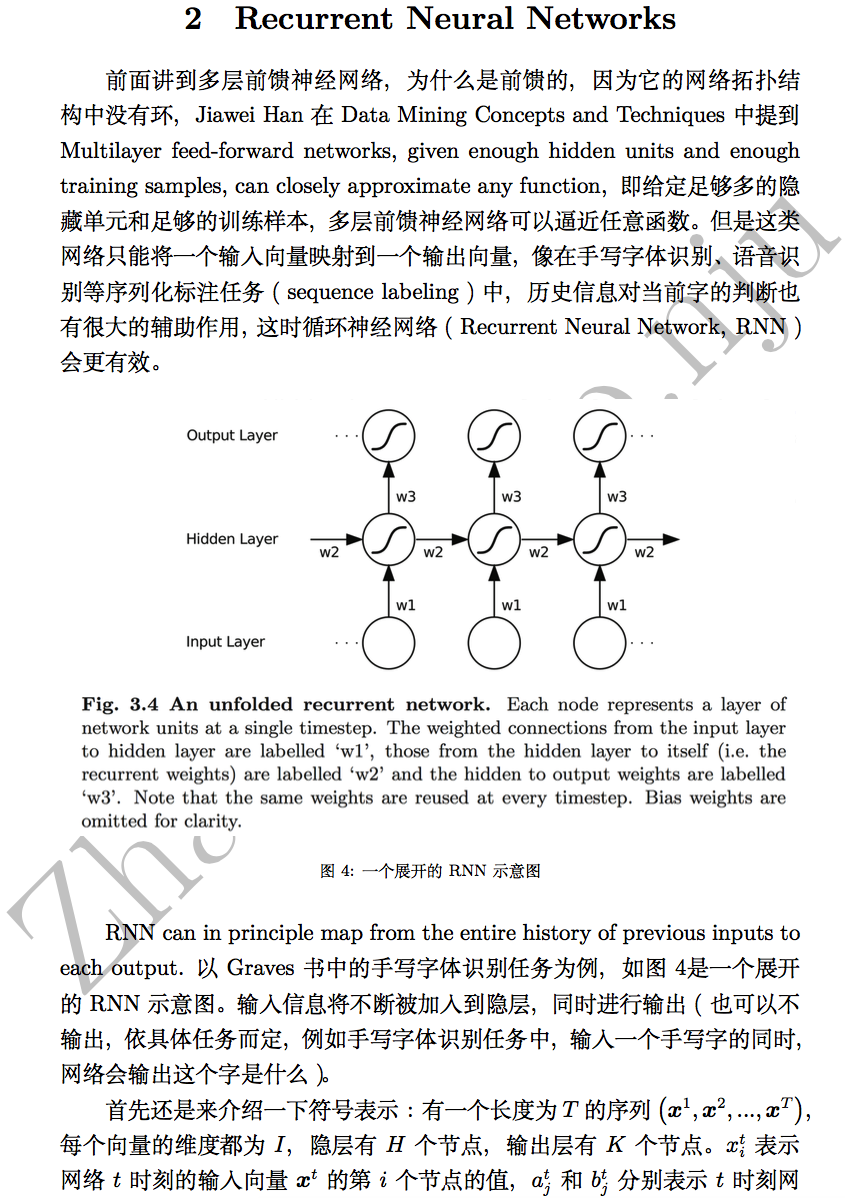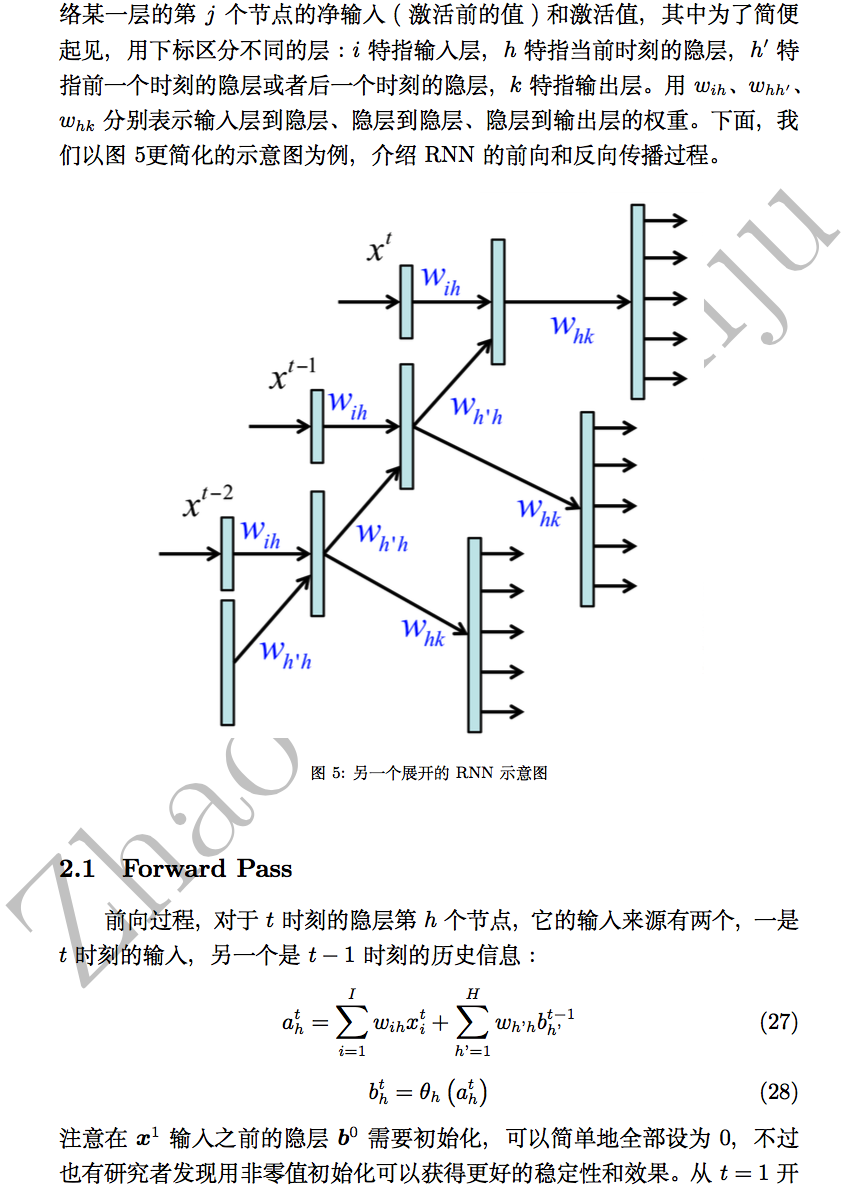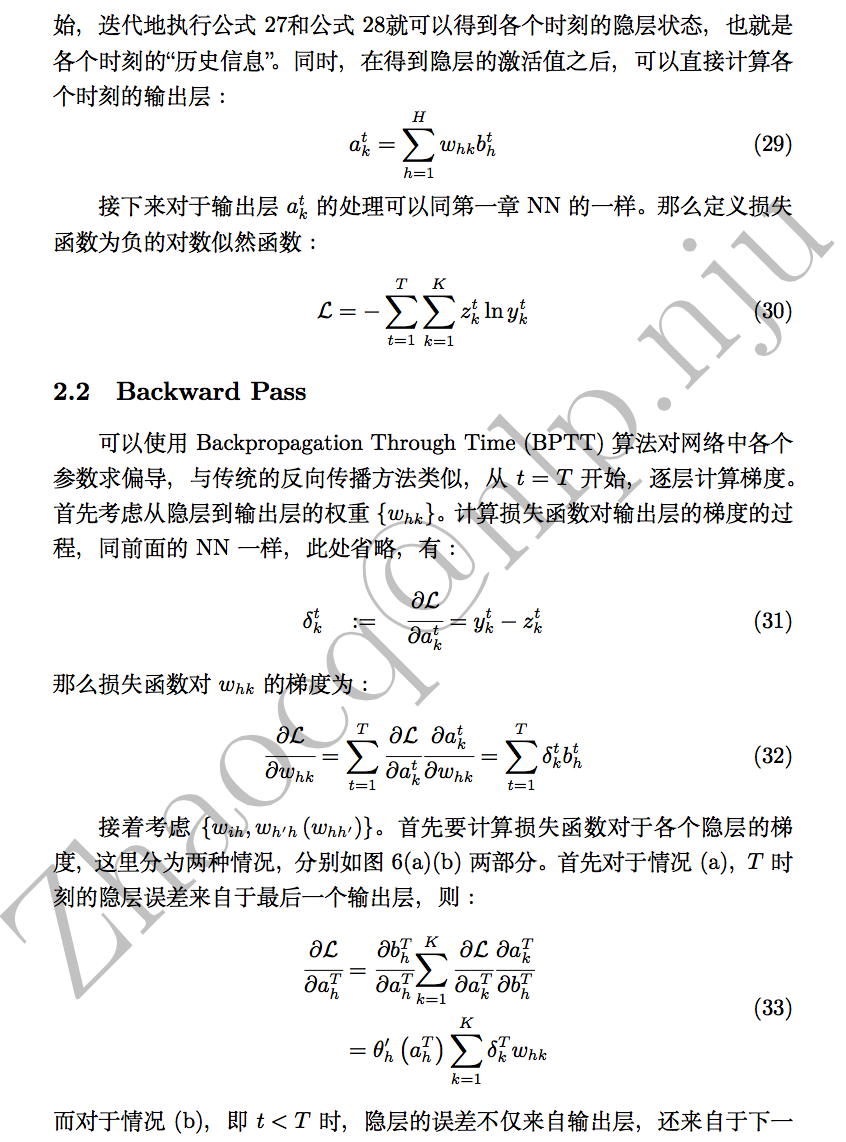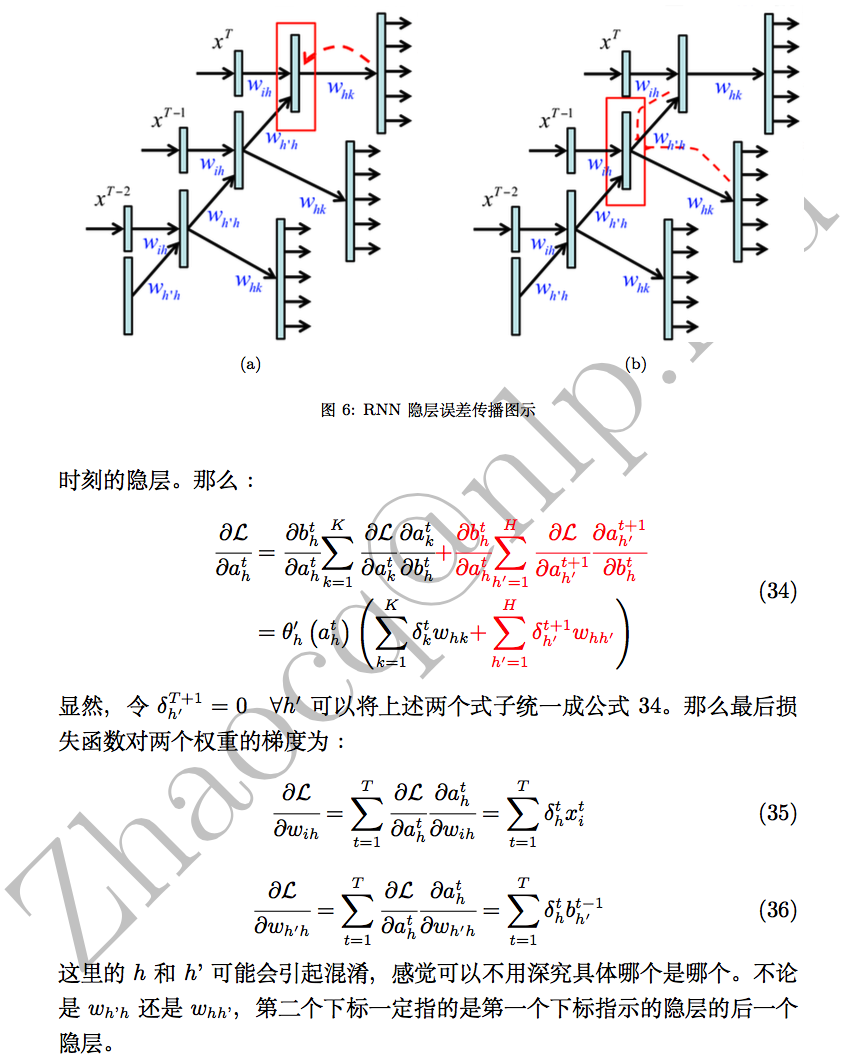从NN到RNN再到LSTM(3): 长短时记忆LSTM简介及计算

本文将简要介绍RNN存在的梯度消失和梯度爆炸问题，然后介绍长短时记忆（Long Short-Term memory，LSTM）的相关公式及推导过程。

转载请注明出处：http://blog.csdn.net/u011414416/article/details/46724699
以下内容主要引自Alex Graves写的Supervised Sequence Labelling with Recurrent Neural Networks 一书。  （http://www.springer.com/cn/book/9783642247965）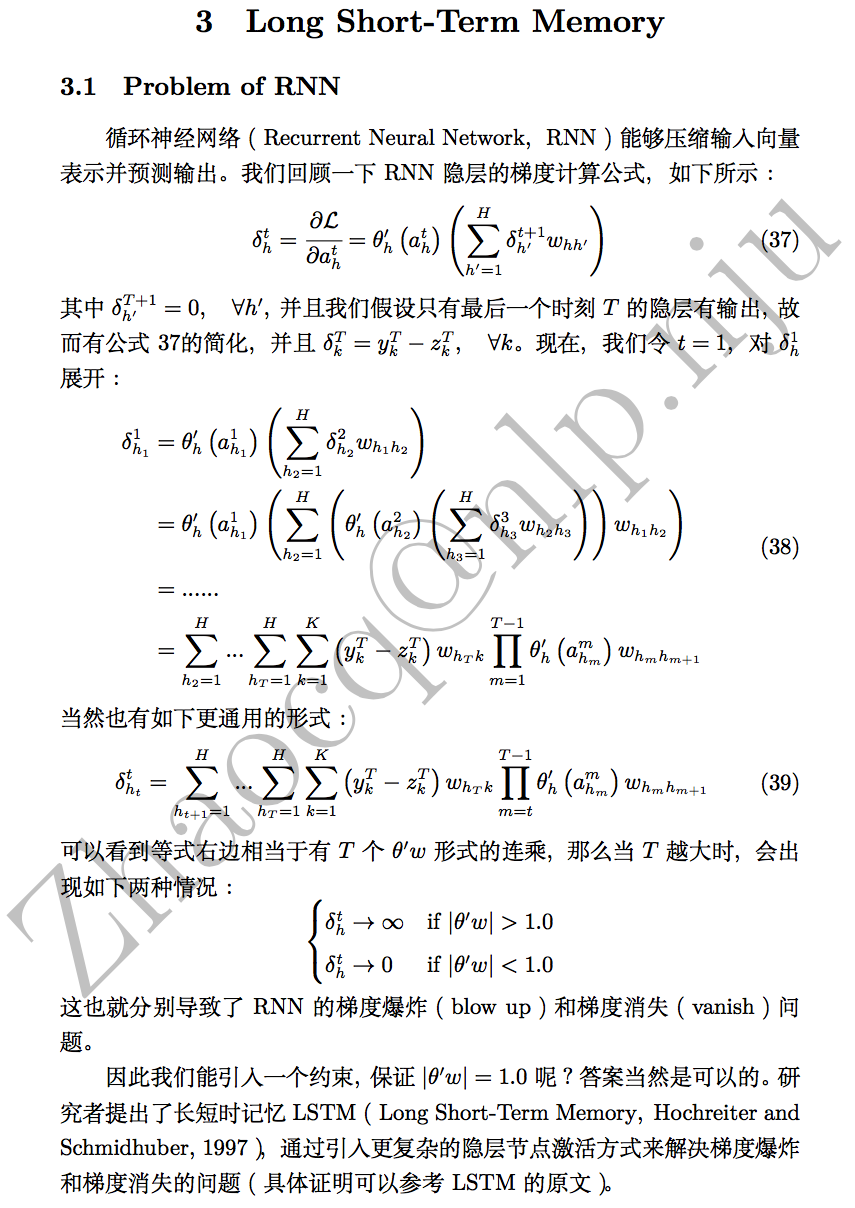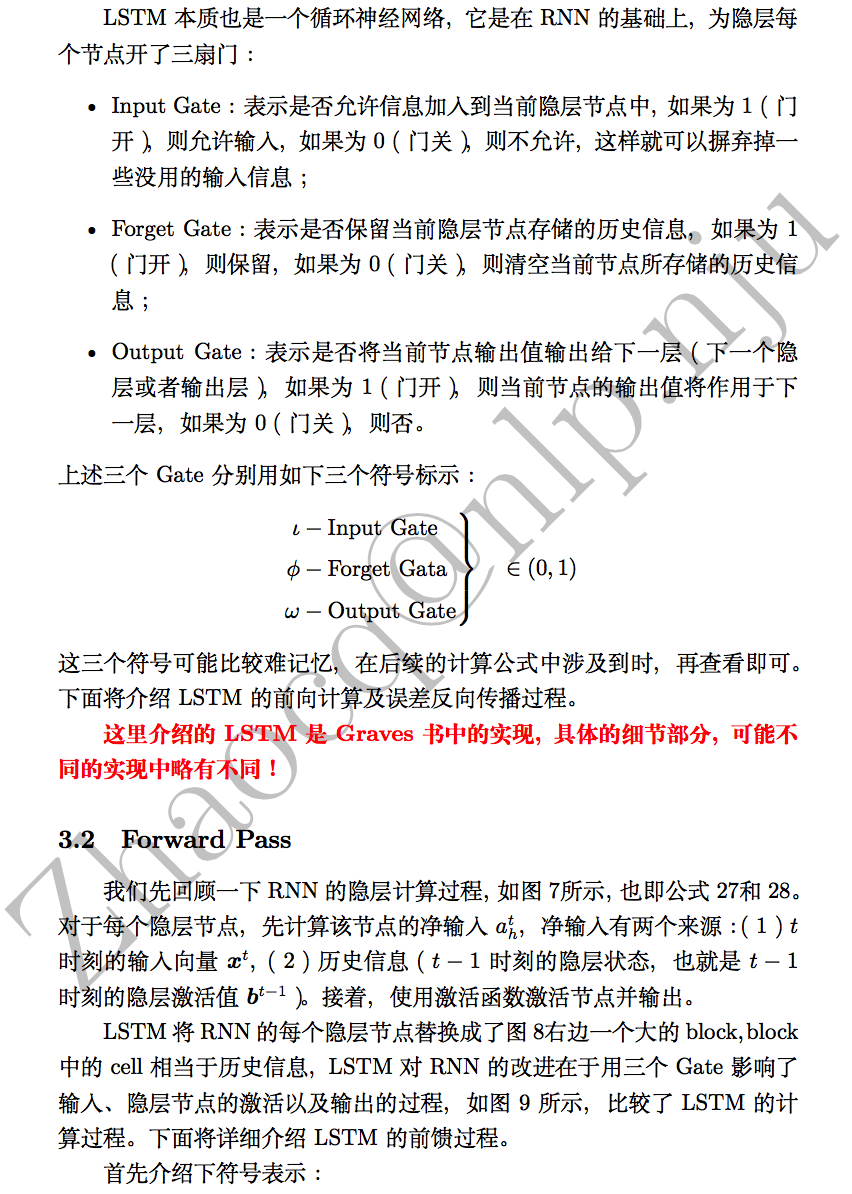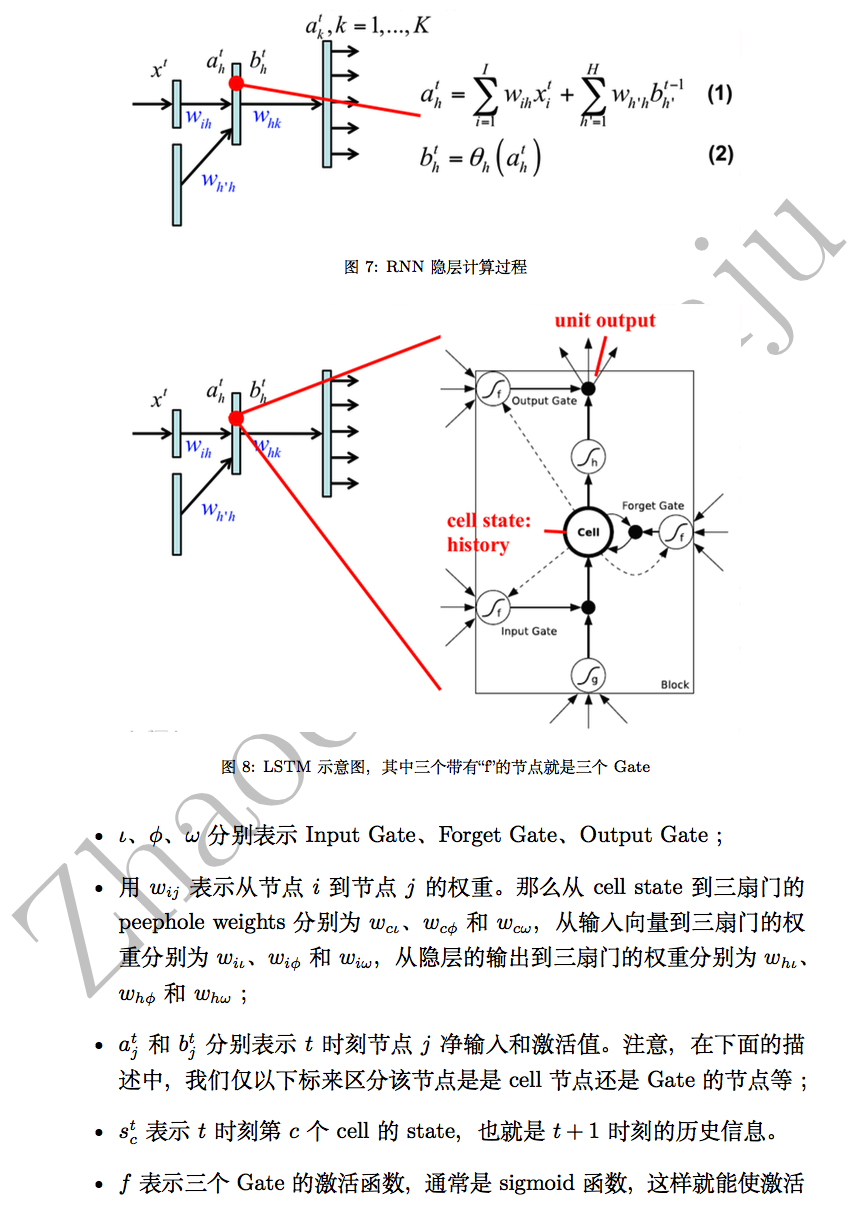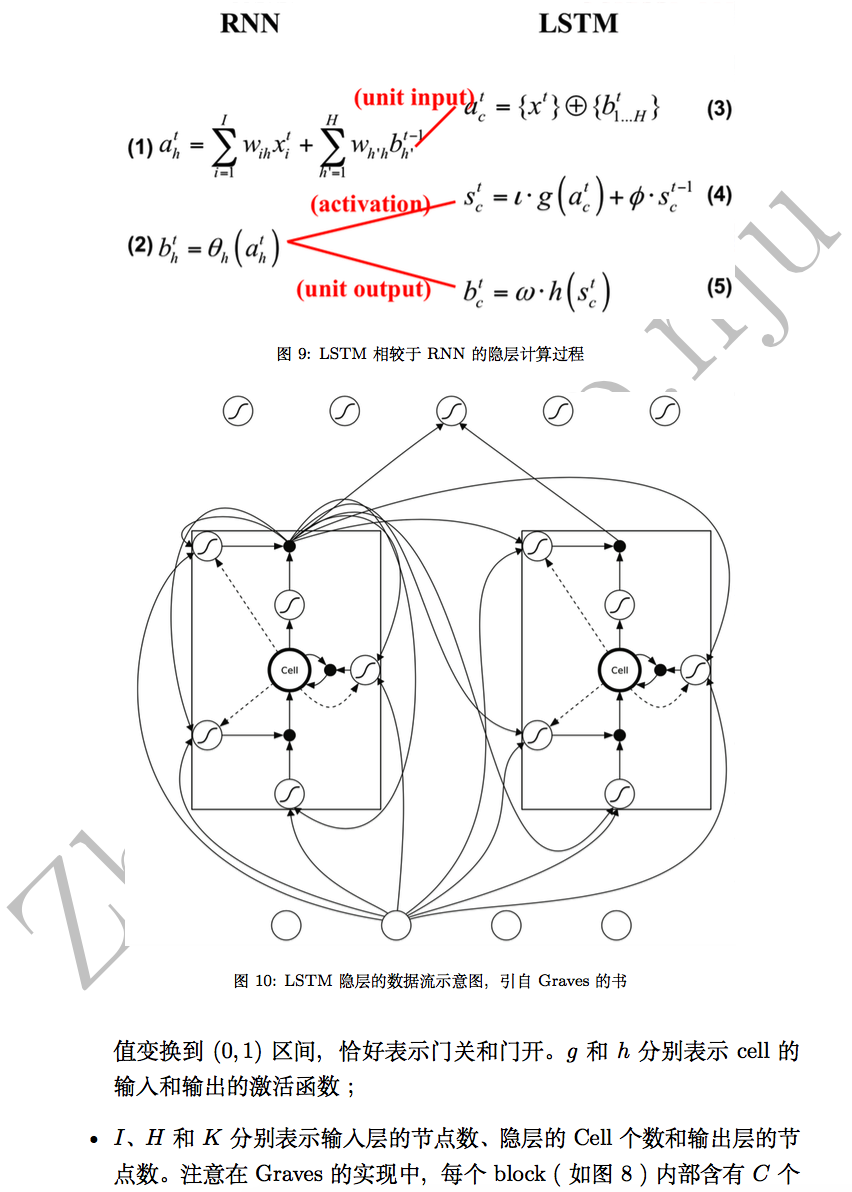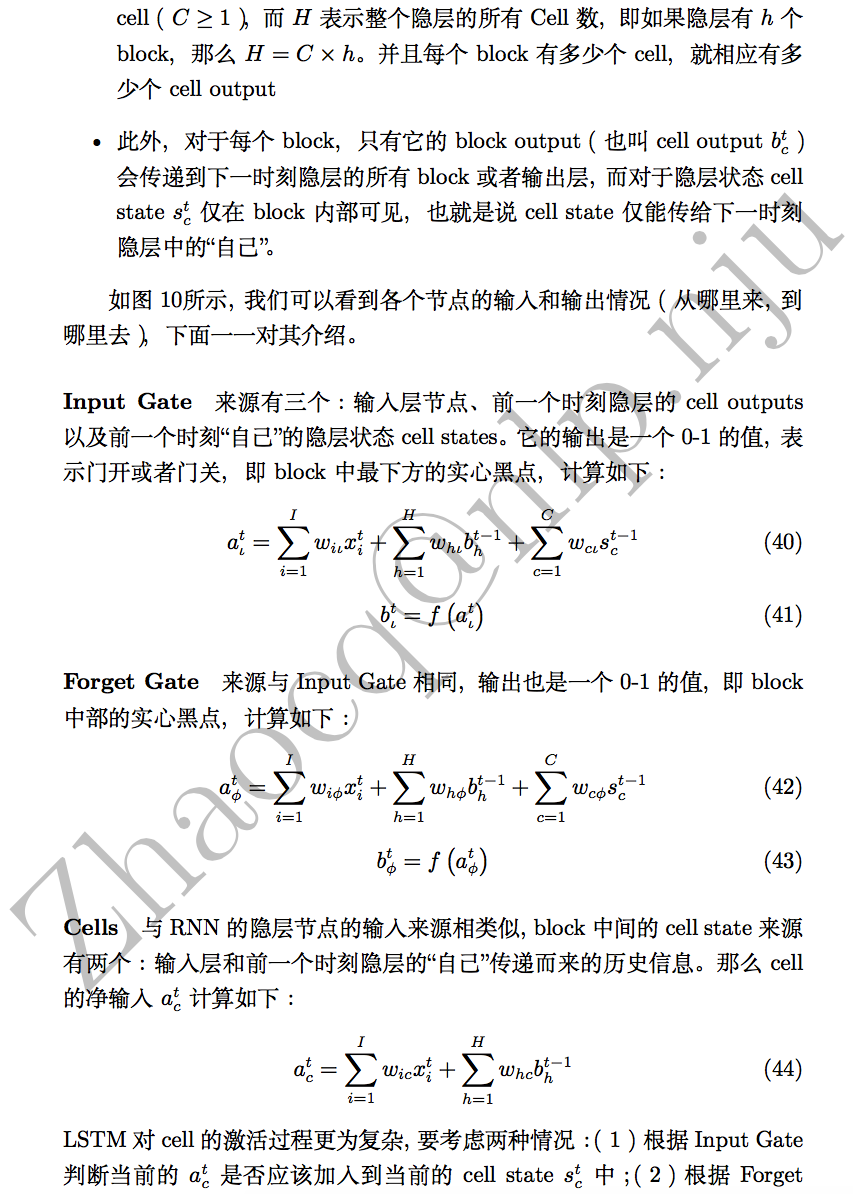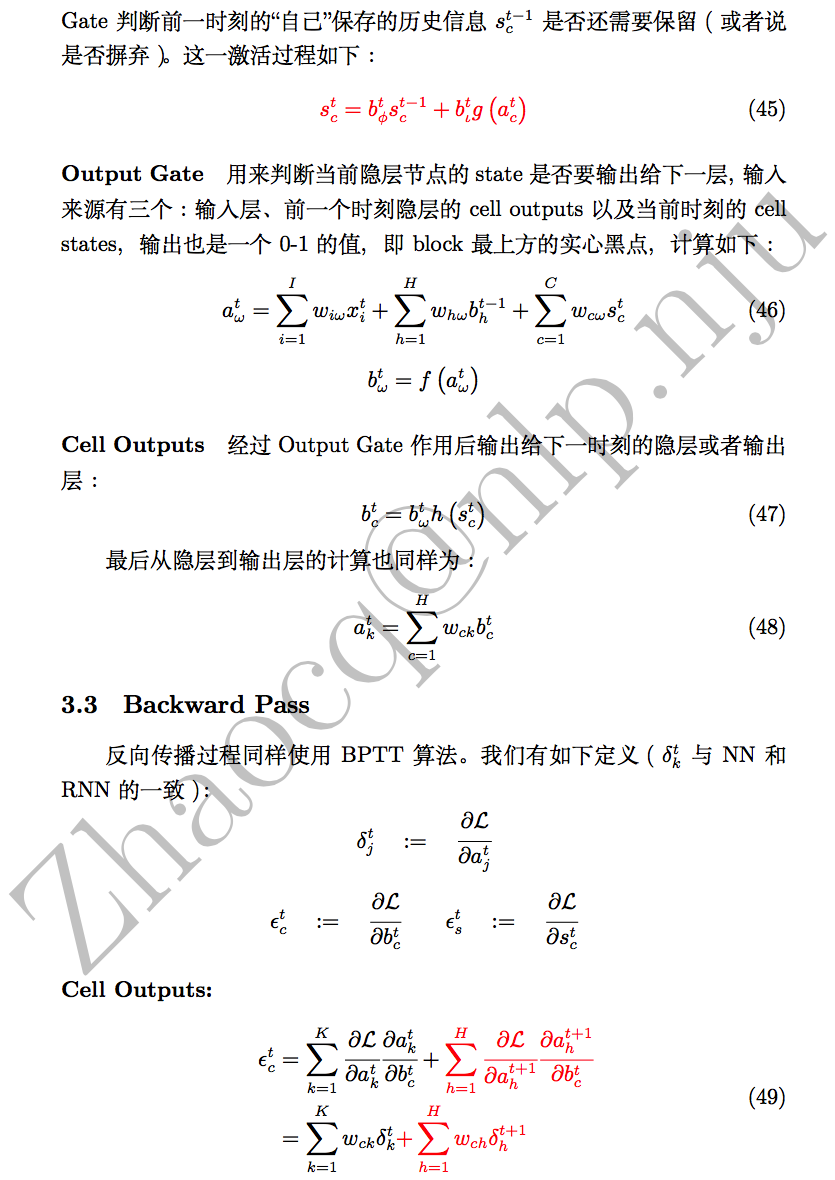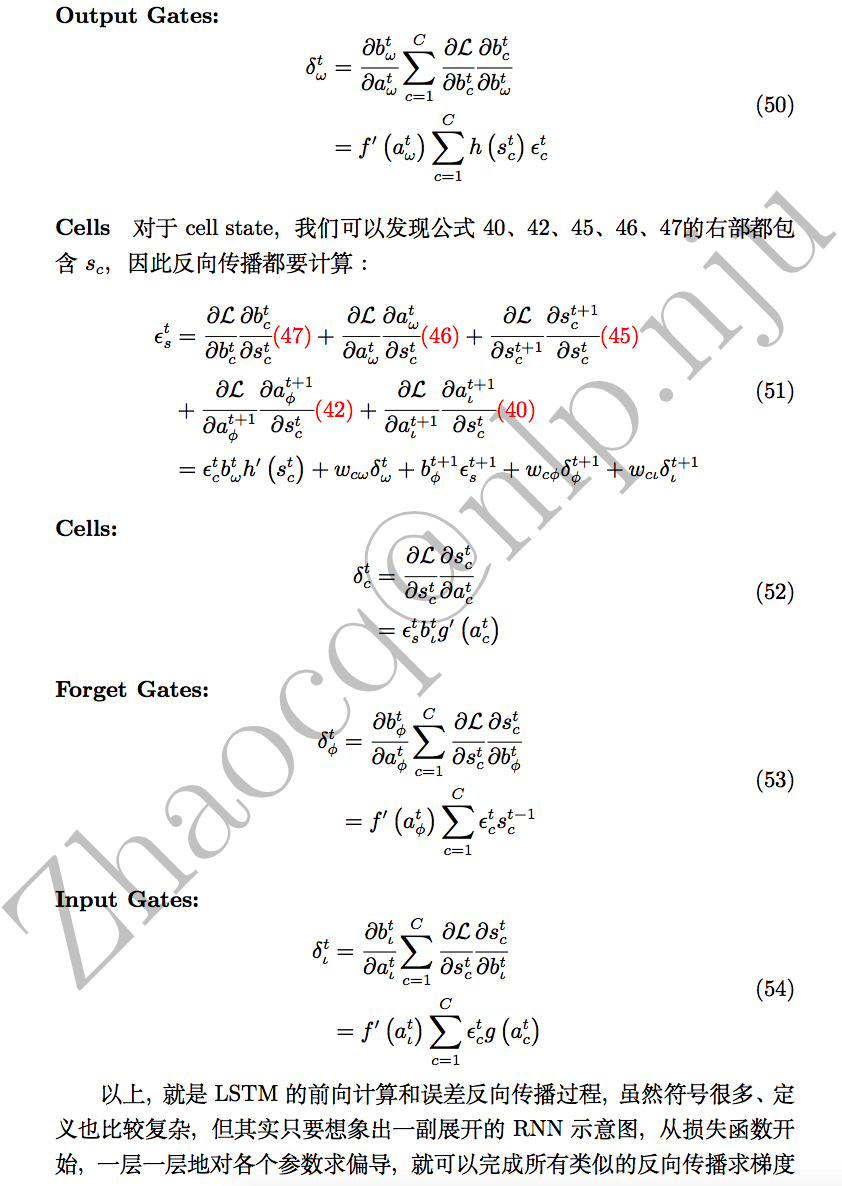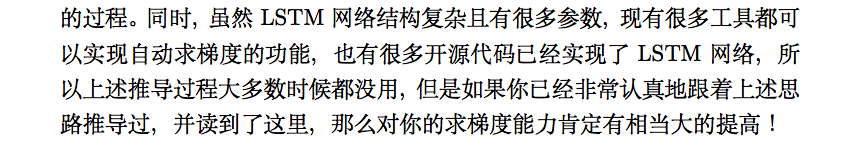展开全文RNNLSTM
• 演示BP神经网络计算过程，反向传播的计算过程，帮助理解神经网络工作原理，算一遍就能懂。
神经网络算法是很早就提出的一种方法，挺起来很高大上，其实说白了就是通过各种数据变换，把输入数据和输出数据建立一种联系。如果是函数的拟合，那么这个联系可以理解为一种复杂的函数关系，如果是分类可以理解通过维度变换以及函数变换找到一个能区分两类数据的超平面（多维）。神经网络方法就是通过建立训练数据与目标的联系对新的输入参数进行预测的过程。
BP神经网络是一种按误差反向传播(简称误差反传)训练的多层前馈网络，其算法称为BP算法，它的基本思想是梯度下降法，利用梯度搜索技术，以期使网络的实际输出值和期望输出值的误差均方差为最小。
整个过程为，首先进行一次正向传播：输入层---->隐含层-->输出层，根据对比输出结果和目标的误差，反向计算层传递权值的误差，调整权值，再进行一次正向传播，反复迭代，实现目标拟合。
以2个参数的三层网络为例子， 通过手动计算一遍整BP神经网络的参数传递过程，来理解整神经网络原理。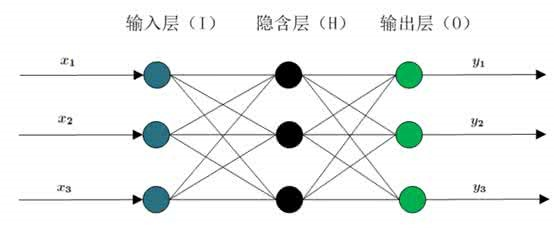三层BP神经网络结构模型
为了简化计算，将模型进行简化，输入层只有
2
个数据点，隐含层和输出层均为两个节点，结构图变为。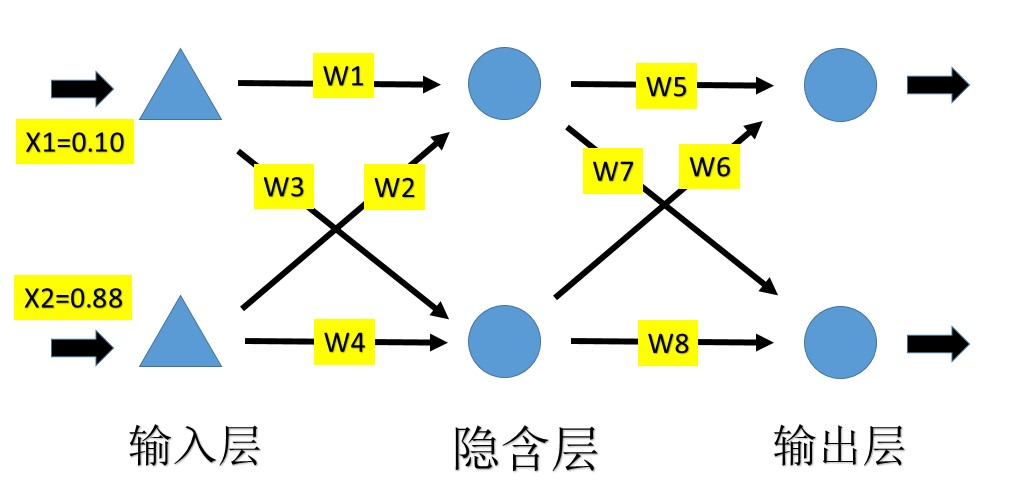输入数据为x1=0.10，x2=0.88，
目标值为：y1=0.55,y2=1
相互之间的权值全部初始为w1-w8=1
正向传递的公式为： y和x是向量，将式子简化令a=1,b=1,正向传播简化为：y=wx
正向传播：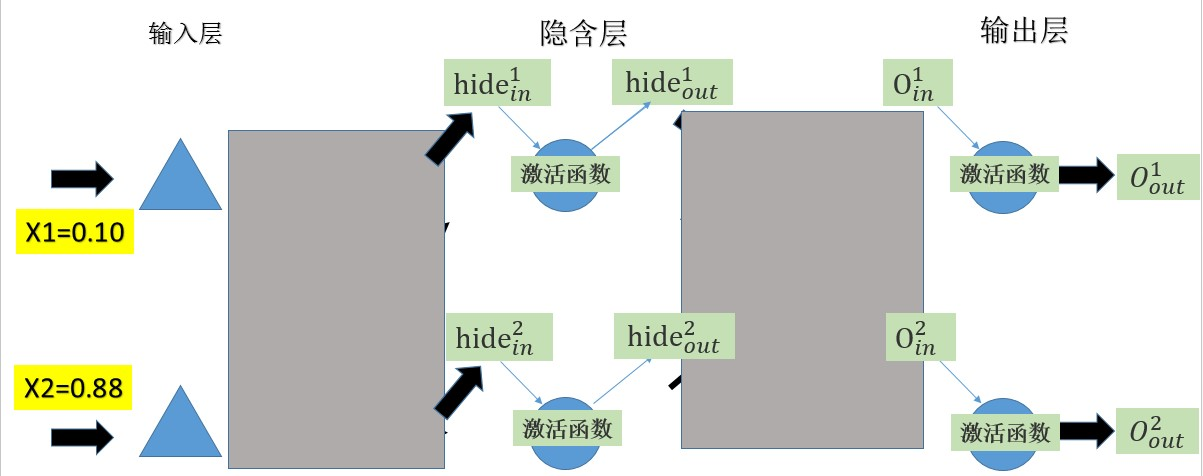隐含层部分（包含输出部分以及激活函数的输出）：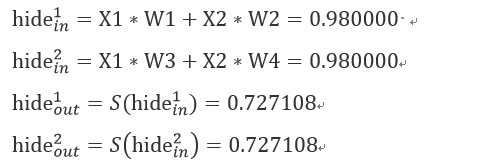输出层部分（包含输出部分以及激活函数的输出）：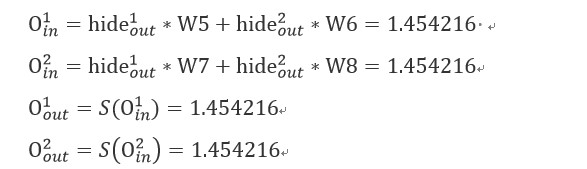正向传播完成,计算损失函数：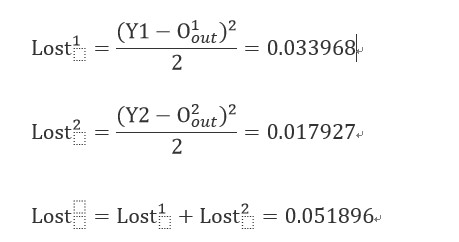反向传播：

计算
Lost
对
w5
，
w6
，
w7
，
w8
（隐含层
—>
输出层）的偏导数，这几个权重对最终误差产生的影响
,
（注意
s
（
x
）的导数）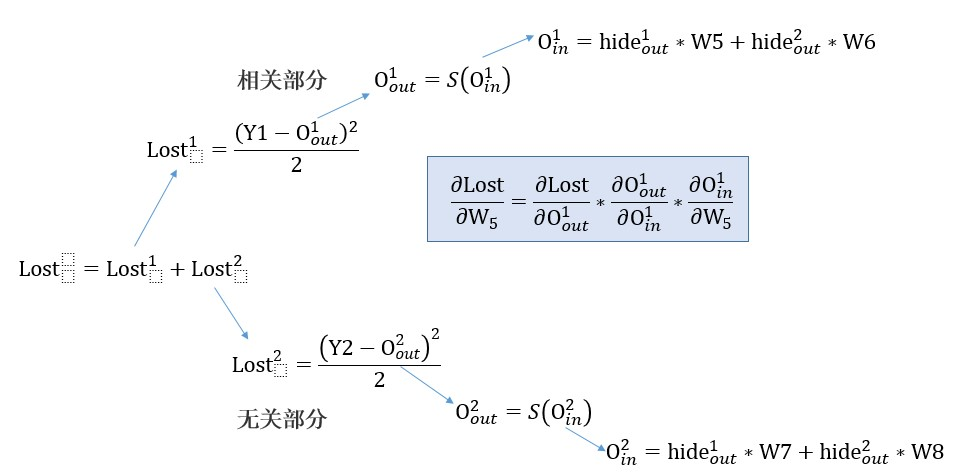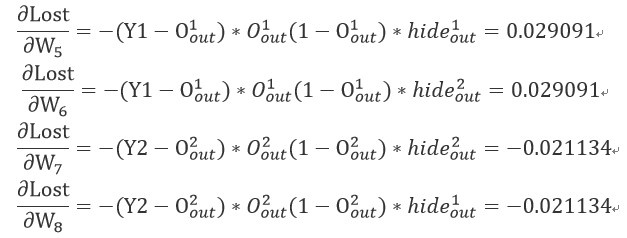输入层—>隐含层）
计算Lost对w1，w2，w3，w4的偏导数,这一层参数同时影响两个输出结果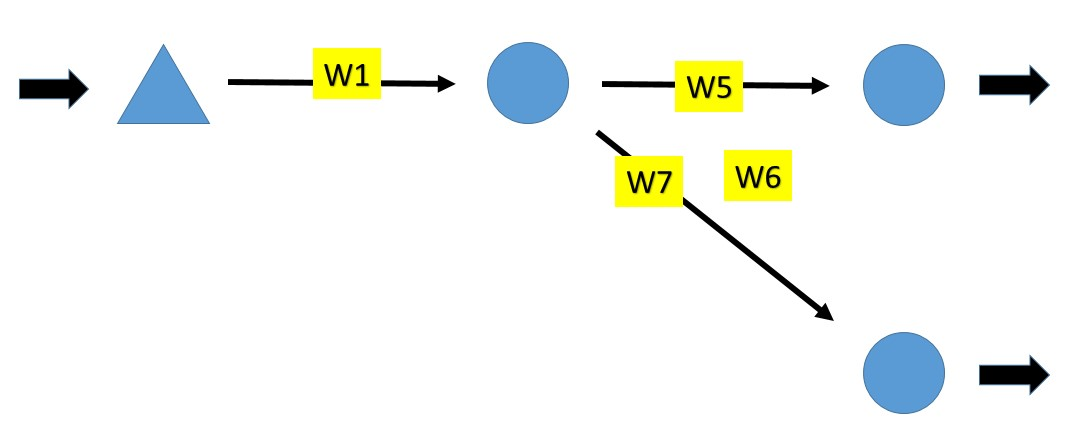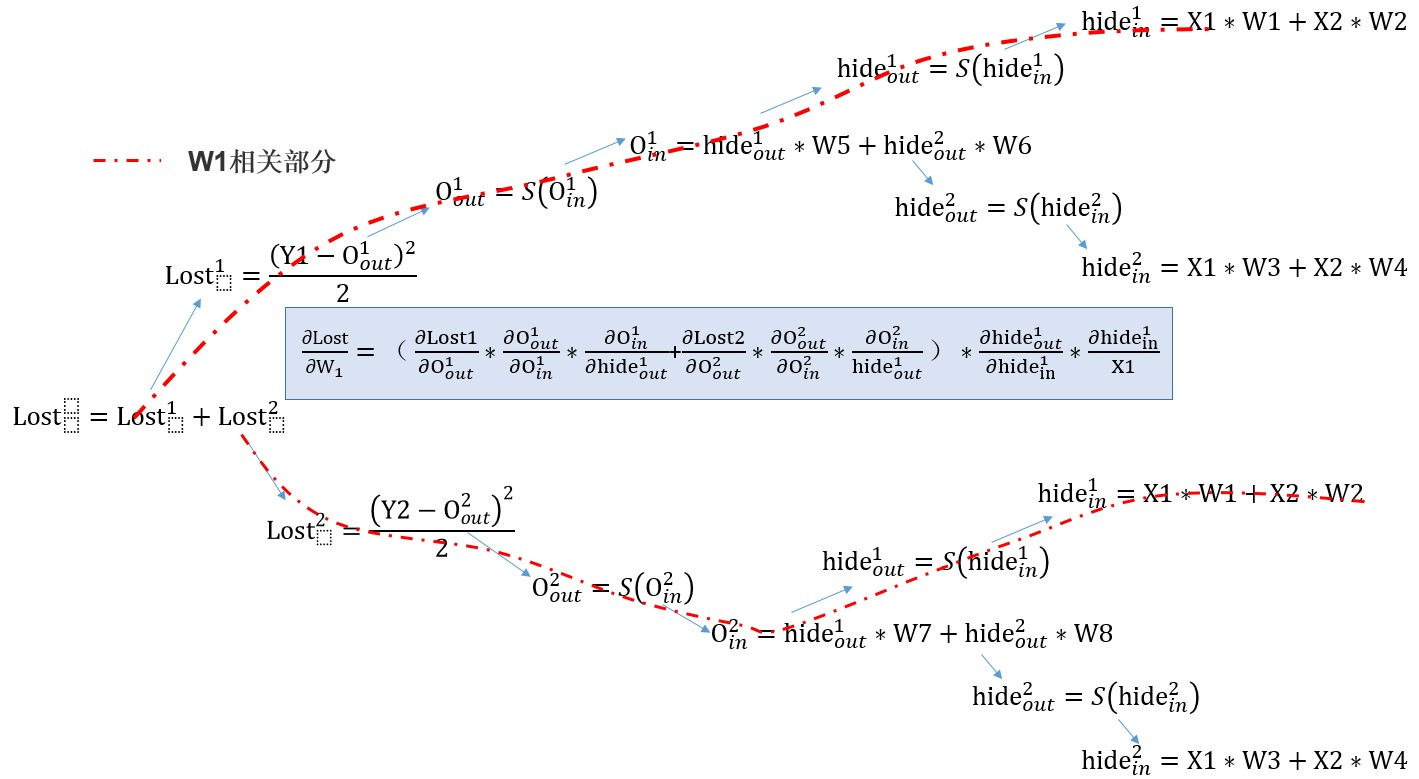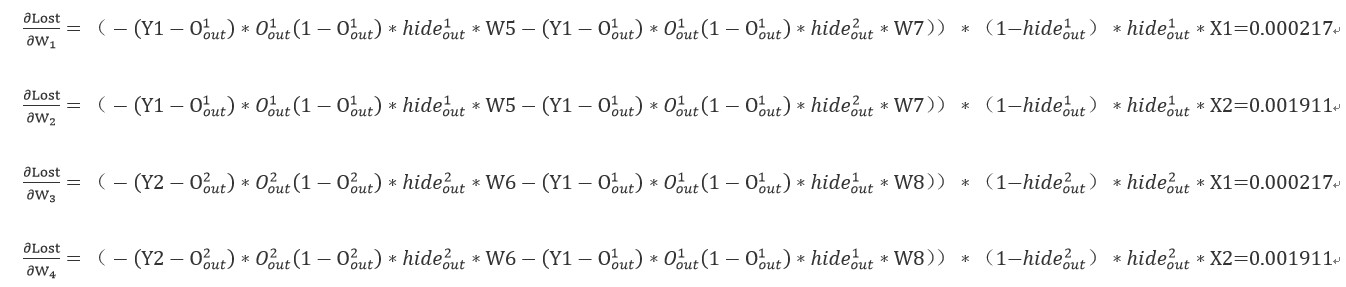调整权值：

梯度下降方法不断优化参数：θ为下降速率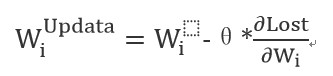第一次传播后lost=0.051896,Y1_=0.81064649903,Y2=0.81064649903

迭代10次后，lost=0.0409254668911,迭代100次后lost=0.00504936047507，
迭代1000次后lost=0.000467508796192，输出结果为Y1_=0.55002398472,Y2=0.969421952039,基本拟合
附上Python的计算过程代码，帮助理解计算过程

import numpy as np
def sigmoid(inX):
return 1.0/(1+np.exp(-inX))
x1 = 0.1
x2 = 0.88
y1 = 0.55
y2 = 1
w1,w2,w3,w4,w5,w6,w7,w8 =1.0,1.0,1.0,1.0,1.0,1.0,1.0,1.0
for i inrange(1):
#-------------开始计算
h_in_1 = w1*x1+w2*x2
h_in_2 = w3*x1+w4*x2
#h_in为传递到隐层的值
print('h_in_1 = %f,h_in_2 = %f'%(h_in_1,h_in_2))

h_out_1 = sigmoid(h_in_1)
h_out_2 = sigmoid(h_in_2)
#h_out从隐层传出的值
print('h_out_1 = %f,h_out_2 = %f'%(h_out_1,h_out_2))

o_in_1 = w5*h_out_1+w6*h_out_2
o_in_2 = w7*h_out_1+w8*h_out_2
#o_in为传入输出层的值
print('o_in_1 = %f,o_in_2 = %f'%(o_in_1,o_in_2))

o_out_1 = sigmoid(o_in_1)
o_out_2 = sigmoid(o_in_2)
#y1,y2为输出的结果
print('o_out_1 = %f,o_out_2 = %f'%(o_out_1,o_out_2))

lost1 = (y1-o_out_1)**2/2
lost2 = (y2-o_out_2)**2/2
lost = lost1+lost2
print('lost = %f'%(lost))

diff_w5 = -(y1-o_out_1)*o_out_1*(1-o_out_1)*h_out_1
diff_w6 = -(y1-o_out_1)*o_out_1*(1-o_out_1)*h_out_2
diff_w7 = -(y2-o_out_2)*o_out_2*(1-o_out_2)*h_out_2
diff_w8 = -(y2-o_out_2)*o_out_2*(1-o_out_2)*h_out_1

diff_w1 = (-(y1-o_out_1)*o_out_1*(1-o_out_1)*w5-(y2-o_out_2)*o_out_2*(1-o_out_2)*w7)*(1-h_out_1)*h_out_1*x1
diff_w2 = (-(y1-o_out_1)*o_out_1*(1-o_out_1)*w5-(y2-o_out_2)*o_out_2*(1-o_out_2)*w7)*(1-h_out_1)*h_out_1*x2
diff_w3 = (-(y2-o_out_2)*o_out_2*(1-o_out_2)*w6-(y1-o_out_1)*o_out_1*(1-o_out_1)*w8)*(1-h_out_2)*h_out_2*x1
diff_w4 = (-(y2-o_out_2)*o_out_2*(1-o_out_2)*w6-(y1-o_out_1)*o_out_1*(1-o_out_1)*w8)*(1-h_out_2)*h_out_2*x2
print('diff_w5 = %f, diff_w6 = %f, diff_w7 = %f, diff_w8= %f'%(diff_w5, diff_w6, diff_w7, diff_w8))
print('diff_w1 = %f, diff_w2 = %f, diff_w3 =%f, diff_w4 = %f'%(diff_w1, diff_w2, diff_w3, diff_w4))
#diff_w为w对代价函数的偏导数

theta = 0.5
update_w5 = w5-theta*diff_w5
update_w6 = w6-theta*diff_w6
update_w7 = w7-theta*diff_w7
update_w8 = w8-theta*diff_w8
update_w1 = w1-theta*diff_w1
update_w2 = w2-theta*diff_w2
update_w3 = w3-theta*diff_w3
update_w4 = w4-theta*diff_w4

print('update_w5 = %f, update_w6 = %f, update_w7 =%f, update_w8 = %f'%(update_w5,update_w6,update_w7,update_w8))
print('update_w1 = %f, update_w2 = %f, update_w3 =%f, update_w4 = %f'%(update_w1,update_w2,update_w3,update_w4))
w5 = update_w5
w6 = update_w6
w7 = update_w7
w8 = update_w8
w1 = update_w1
w2 = update_w2
w3 = update_w3
w4 = update_w4
print(lost)
print(o_out_1, o_out_2)

运行结果：h_in_1 = 0.980000,h_in_2 = 0.980000
h_out_1 = 0.727108,h_out_2 = 0.727108
o_in_1 = 1.454216,o_in_2 = 1.454216
o_out_1 = 0.810646,o_out_2 = 0.810646
lost = 0.051896
diff_w5 = 0.029091,diff_w6 = 0.029091,diff_w7 = -0.021134,diff_w8 = -0.021134
diff_w1 = 0.000217,diff_w2 = 0.001911,diff_w3 = 0.000217,diff_w4 = 0.001911
update_w5 = 0.985455,update_w6 = 0.985455,update_w7 = 1.010567,update_w8 = 1.010567
update_w1 = 0.999891,update_w2 = 0.999045,update_w3 = 0.999891,update_w4 = 0.999045
0.0518956728931
0.81064649903 0.81064649903


展开全文神经网络 数据分析 python 实例
• 下面将以目前流行的计算图的方式，展示一个神经网络的计算，是如何按照前向或反向传播过程组织起来的。 1、计算图（Computation Graph）的计算 尝试计算函数J，J是由三个变量a,b,c组成的函数，这个函数是3(a+bc)...
在上两篇的学习笔记中，学习了数据集的表示和处理、激活函数的选取、代价函数的设计技巧和梯度下降的过程，那么神经网络在下降的计算过程是怎样的呢？下面将以目前流行的计算图的方式，展示一个神经网络的计算，是如何按照前向或反向传播过程组织起来的。
1、计算图（Computation Graph）的计算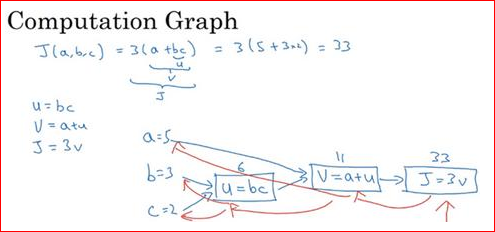尝试计算函数J，J是由三个变量a,b,c组成的函数，这个函数是3(a+bc) 。计算这个函数走三个步骤，首先计算 b 乘以 c，把它储存在变量u中，u=bc； 然后计算v=a+u；最后输出J=3v，这就是要计算的函数J。可以把这三步画成如上的计算图，先画三个变量a,b,c，第一步就是计算u=bc，在这周围放个矩形框，它的输入是b,c，接着第二步v=a+u，最后一步J=3v。
举个例子: a=5,b=3,c=2 ，u=bc就是6，就是5+6=11。J是3倍的v ，因此即3×(5+3×2)。如果把它算出来，实际上得到33就是J的值。 当有不同的或者一些特殊的输出变量时，例如本例中的J和逻辑回归中想优化的代价函数J，因此计算图用来处理这些计算会很方便。从这个小例子中可以看出，通过一个从左向右的过程，你可以计算出J的值。
为了计算导数，从右到左（红色箭头，和蓝色箭头的过程相反）的过程是用于计算导数最自然的方式。计算图组织计算的形式是用蓝色箭头从左到右的计算，下面看看如何进行反向红色箭头(也就是从右到左)的导数计算

2、计算图的导数计算
先来张计算图的计算：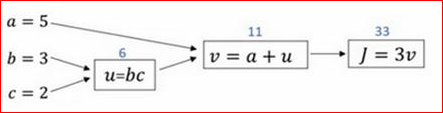假设要计算dJ/dv，那要怎么算呢？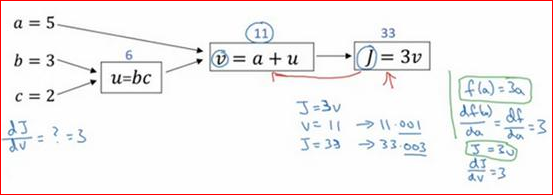其实dJ/dv和上一篇的学习笔记中的导数描述如出一辙，看 如上图的增量三角的描述。它的斜率是3。在反向传播算法中，如果想计算最后输出变量的导数，使用最关心的变量对v的导数，那么就做完了一步反向传播，在这个流程图中是一个反向步。
dJ/da是多少呢？换句话说，如果我们提高a的数值，对J的数值有什么影响？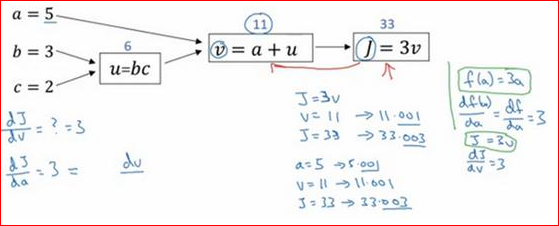在变量a=5时，让它增加到5.001，那么对v的影响就是a+u,之前v=11，现在变成11.001，从上图看到现在J就变成33.003了，所以如果让a增加0.001，J增加0.003。如果把这个5换成某个新值，那么a的改变量就会传播到流程图的最右端，所以J的增量是3乘以a的增量，意味着这个导数是3。要解释这个计算过程，其中一种方式是：如果改变了a，那么也会改变v，通过改变v，也会改变J。所以J值的净变化量就是：当提升这个值（0.001），当把a值提高一点点，这就是J的变化量（0.003）。
首先a增加了，v也会增加，v增加多少呢？这取决于dv/da，然后v的变化导致J也在增加，所以这在微积分里实际上叫链式法则，如果a影响到v，v影响到J，那么当让a变大时，J的变化量就是当改变a时，v的变化量乘以改变v时J的变化量，在微积分里这就叫链式法则。
数学描述这个计算：dJ/da=（dJ/dv）*（dv/da），所以这个乘积就是3×1，实际上dJ/da=3。（至于dv/da这个偏导大家自行解算哈）。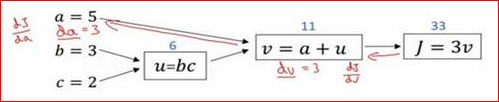上图完成部分的反向传播算法dJ/da，这里约定dJ/da简述da，dJ/dv简述dv。这个新的符号约定，就是当编程实现反向传播时，通常会有一个最终输出值是要关心的，最终的输出变量，真正想要关心或者说优化的。那接下来按照以上的这个套路算算其余的导数/反向传播过程。
du=dJ/du=（dJ/dv）*（dv/du）=3×1: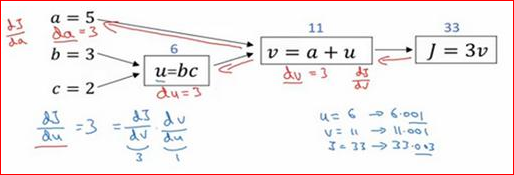db=dJ/db=（dJ/dv）*（dv/du）*（du/db）=3×1×2:(例子中c=2)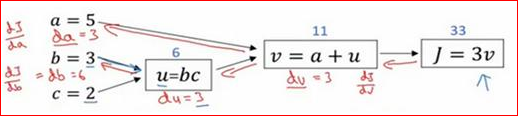dc=dJ/dc=（dJ/dv）*（dv/du）*（du/dc）=3×1×3:(例子中b=3)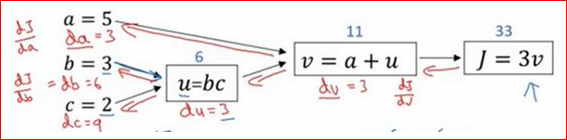可以说，一个神经网络的计算，都是按照前向或反向传播过程组织的。首先计算出一个新的网络的输出（前向过程），紧接着进行一个反向传输操作。所以这是一个计算流程图，就是正向或者说从左到右的计算来计算成本函数J或是可能需要优化的函数，然后反向从右到左计算导数。如果熟悉了微积分或链式法则，就知道需要做什么才能编写代码，实现逻辑回归模型中的导数计算。
下一篇的学习笔记便是编写代码，通过逻辑回归的实现来表达下此篇以及前两篇中有关数据集如何表示和处理、选用的激活函数、成本函数和此篇的前向传播和反向传播的过程，梯度下降又是如何起作用的。
展开全文神经网络 前向传播
• 计算图的反向传播 链式法则和计算图 加法节点的反向传播 乘法节点的反向传播 “购买水果”问题的反向传播 激活函数（层）的反向传播 激活函数ReLU的反向传播 激活函数Sigmoid的反向传播 Affine/softmax激活...深度学习 神经网络 权重参数 梯度
• 以激活函数f为ReLU，损失函数为softmax的交叉熵损失的3-layer神经网络为例，给出完整的计算各层梯度的过程（下图中，W3的 size 应该是 [H,C]）：
• 计算计算图就是将计算过程用图形表示出来，这里所说的图形是数据结构图，通过多个节点和边表示（边是用来连接节点的）。 下面我们先来通过一个简单的例子了解计算图的计算过程 假设我们有如下需求： 一个苹果100...深度学习
• 计算图的正向传播与反向传播 计算计算图就是一种表示计算过程的数据结构图。计算图通过节点表示某种运算，用带箭头的边表示运算的方向，箭头上标有与运算相关的数据。上图就是一张计算图。 正向传播 上图中...
• 分析：一般来讲，对于数值n，其二进制数的最后一位是n % 2，因此计算出的第一个数字恰好是需要输出的最后一位数字。我们使用一个递归函数实现，在函数中，首先在递归调 用之前计算n % 2的数值，然后在递归调用语句...C 递归
• 通过上一讲3D-2D的位姿计算后，现在求取反向投影误差 首先通过cv::solvePnPRansac(pts3d,pts2d,K,Mat(),rvec,tvec,false,100,4.0,0.99,inliers);cv::solvePnPRansac( pts3d, pts2d, K, Mat(), rvec, tvec, false, ...函数
• 今天学习pytorch实现简单的线性回归，发现了pytorch的反向传播时计算梯度采用的累加机制， 于是百度来一下，好多博客都说了累加机制，但是好多都没有说明这个累加机制到底会有啥影响， 所以我趁着自己练习的一个例子...
• 1.反向传播的目的 目的就是计算权重、偏置等超参数的梯度，方便用优化算法更新参数时用到 2.为什么要从后向前计算梯度 避免重复计算，从而加快计算速度 参考：反向传播算法为什么要“反向” ...
• BP反向传播算法的具体思想和计算方式 BP算法采用有监督学习方式，基于梯度下降算法，极小化误差函数 主要思想：将学习过程分为信号正向传播过程和误差反向传播过程两个阶段。 什么是梯度下降算法？梯度下降算法：...bp算法 神经网络
• Backpropagation算法是目前绝大...的影响，我们就需要进行多次计算，所以正向微分算法在效率上明显不如反向微分，这也是我们选择反向微分算法的原因。 参考： Calculus on Computational Graphs: Backpropagation神经网络
• CNN进行前向传播阶段点，依次调用每个Layer的Forward函数，得到逐层的输出，最后一层与目标函数比较得到损失函数，计算误差更新值，通过反向传播路径层达到第一层，所有的权值层在反向传播结束后一起更新。...
• 本文主要内容：2.1 误差的计算2.1.1 示例：2.1.2 Cross Entropy Loss交义熵损失2.1.3 回到前面的示例2.2 误差的反向传播2.3 权重的更新 2.1 误差的计算 2.1.1 示例： 下面以一个三层的BP神经网络为例进行讲述，如下...人工智能 深度学习 神经网络
• 很早以前做单片机时，知道马达，继电器一类电感线圈需要并联一个反向续流二极管，防止电感产生的反向电动势损坏线圈。近来突发思考，在考虑如何彻底 地理解这个反向电动势的产生及方向问题，期间查阅了相关资料，也...
• 文章目录反向传播算法1.激活函数导数1.1 Sigmoid函数导数1.2 ReLU 函数导数1.3 LeakyReLU函数导数1.4 Tanh 函数梯度2.链式法则3.反向传播算法实战 反向传播算法 1.激活函数导数 1.1 Sigmoid函数导数 Sigmoid函数...深度学习 神经网络 tensorflow 机器学习
• 计算图 用计算图来表示任何函数，其中图的节点表示我们要执行的每一步计算。如上图的线性分类器中，输入是xxx和WWW，∗*∗表示矩阵乘法，即W∗xW*xW∗x，输出得分向量。另一个节点表示 hinge loss，计算数据损失项...深度学习 线性代数
• 前向传递节点，反向传递梯度。 我们先从计算图的底部开始向上，逐个节点计算函数值并保存下来。这个步骤，叫做前向计算(forward)。 然后，我们从计算图的顶部开始向下，逐步计算损失函数对每个子节点的导函数，代入...
• 神经网络中用反向传播求偏导数的思想就相当于复合函数求偏导。 从头说起，在学生时代我们要求下面式子中，函数e(a,b)e(a,b)e(a,b)对a和b的偏导数： e=(a+b)∗(b+1)e=(a+b)*(b+1)e=(a+b)∗(b+1) ∂e∂a=?\frac{\...神经网络 pytorch 机器学习
• NN的学习中需要计算权重和偏置参数的梯度，对于梯度的计算，很容易想到数值导数，即前向差分 dx=f(x+h)−f(x)hdx=\frac{f(x+h)-f(x)}{h}dx=hf(x+h)−f(x)​ 或者改进一点，用中心差分(更接近准确的导数值) dx=f(x+h)...python...